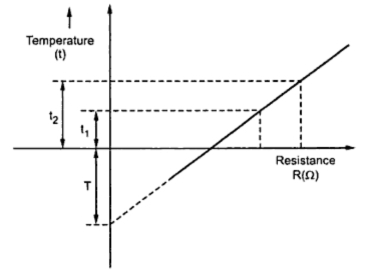### Resistance

The resistance of a transmission line is an important parameter as it is the main cause of power loss in a transmission line. It is defined as the opposition offered by the transmission line conductors to the flow of current. The resistance of the line is uniformly distributed along its whole length. But the performance of the line can be conveniently analaysed by considering the distributed resistance as lumped one as shown in the Fig. 1.
The effective resistance of a conductor is given by
Where the power loss in conductor is in watts and current flowing through the conductor in amperes.
When the distribution of current in a conductor is uniform then the effective resistance is equal to d.c. resistance of the conductor. This direct resistance is given by,
where ρ = resistivity of conductor (Ω-m)
l = length of conductor (m)
a = cross-sectional area of conductor (m2)
The d.c. resistance computed from the above equation of the stranded conductors is greater as spiraling of the strands makes them longer than the conductor itself. This increase in resistance is taken as 1% for 3 strand conductors and 2% for concentrically stranded conductors.
Temperature is also one of the factor which will change the d.c. resistance of the metallic conductor. Its variation with resistance is as shown in the Fig. 1. It is seen to be linear over normal range of operation.Fig. 1
From the above Fig. 1 it can be seen that
where R1, R2 are the resistances if the conductor at temperature t1 and t2 in oC and T is the constant obtained from the graph. The value of T is 234.5oC for annealed copper 100% conductivity.